# Tag: quivers

A few years ago a student entered my office asking suggestions for his master thesis.

“I’m open to any topic as long as it has nothing to do with those silly quivers!”

At that time not the best of opening-lines to address me and, inevitably, the most disastrous teacher-student-conversation-ever followed (also on my part, i’m sorry to say).

This week, Markus Reineke had a similar, though less confrontational, experience. Markus gave a mini-course on ‘moduli spaces of representations’ in our advanced master class. Students loved the way he introduced representation varieties and constructed the space of irreducible representations as a GIT-quotient. In fact, his course was probably the first in that program having an increasing (rather than decreasing) number of students attending throughout the week…

In his third lecture he wanted to illustrate these general constructions and what better concrete example to take than representations of quivers? Result : students’ eyes staring blankly at infinity…

What is it that quivers do to have this effect on students?

Perhaps quiver-representations cause them an information-overload.

Perhaps we should take plenty of time to explain that in going from the quiver (the directed graph) to the path algebra, vertices become idempotents and arrows the remaining generators. These idempotents split a representation space into smaller vertex-spaces, the dimensions of which we collect in a dimension-vector, the big basechange group splits therefore into a product of small vertex-basechanges and the action of this product on an matrix corresponding to an arrow is merely usual conjugation by the big basechange-group, etc. etc. Blatant trivialities to someone breathing quivers, but probably we too had to take plenty of time once to disentangle this information-package…

But then, perhaps they consider quivers and their representations as too-concrete-old-math-stuff, when there’s so much high-profile-fancy-math still left to taste.

When given the option, students prefer you to tell them monstrous-moonshine stories even though they can barely prove simplicity of $A_5$, they want you to give them a short-cut to the Langlands programme but have never had the patience nor the interest to investigate the splitting of primes in quadratic number fields, they want to be taught schemes and their structure sheaves when they still struggle with the notion of a dominant map between varieties…

In short, students often like to run before they can crawl.

Working through the classification of some simple quiver-settings would force their agile feet firmly on the ground. They probably experience this as a waste of time.

Perhaps, it is time to promote slow math…

The Monster is the largest of the 26 sporadic simple groups and has order

808 017 424 794 512 875 886 459 904 961 710 757 005 754 368 000 000 000

= 2^46 3^20 5^9 7^6 11^2 13^3 17 19 23 29 31 41 47 59 71.

It is not so much the size of its order that makes it hard to do actual calculations in the monster, but rather the dimensions of its smallest non-trivial irreducible representations (196 883 for the smallest, 21 296 876 for the next one, and so on).

In characteristic two there is an irreducible representation of one dimension less (196 882) which appears to be of great use to obtain information. For example, Robert Wilson used it to prove that The Monster is a Hurwitz group. This means that the Monster is generated by two elements g and h satisfying the relations

$g^2 = h^3 = (gh)^7 = 1$

Geometrically, this implies that the Monster is the automorphism group of a Riemann surface of genus g satisfying the Hurwitz bound 84(g-1)=#Monster. That is,

g=9619255057077534236743570297163223297687552000000001=42151199 * 293998543 * 776222682603828537142813968452830193

Or, in analogy with the Klein quartic which can be constructed from 24 heptagons in the tiling of the hyperbolic plane, there is a finite region of the hyperbolic plane, tiled with heptagons, from which we can construct this monster curve by gluing the boundary is a specific way so that we get a Riemann surface with exactly 9619255057077534236743570297163223297687552000000001 holes. This finite part of the hyperbolic tiling (consisting of #Monster/7 heptagons) we’ll call the empire of the monster and we’d love to describe it in more detail.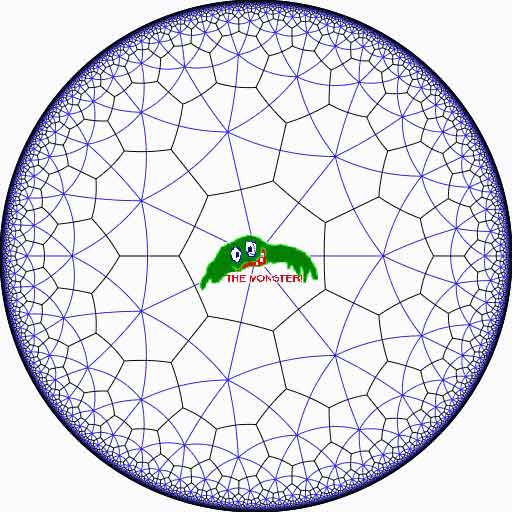Look at the half-edges of all the heptagons in the empire (the picture above learns that every edge in cut in two by a blue geodesic). They are exactly #Monster such half-edges and they form a dessin d’enfant for the monster-curve.

If we label these half-edges by the elements of the Monster, then multiplication by g in the monster interchanges the two half-edges making up a heptagonal edge in the empire and multiplication by h in the monster takes a half-edge to the one encountered first by going counter-clockwise in the vertex of the heptagonal tiling. Because g and h generated the Monster, the dessin of the empire is just a concrete realization of the monster.

Because g is of order two and h is of order three, the two permutations they determine on the dessin, gives a group epimorphism $C_2 \ast C_3 = PSL_2(\mathbb{Z}) \rightarrow \mathbb{M}$ from the modular group $PSL_2(\mathbb{Z})$ onto the Monster-group.

In noncommutative geometry, the group-algebra of the modular group $\mathbb{C} PSL_2$ can be interpreted as the coordinate ring of a noncommutative manifold (because it is formally smooth in the sense of Kontsevich-Rosenberg or Cuntz-Quillen) and the group-algebra of the Monster $\mathbb{C} \mathbb{M}$ itself corresponds in this picture to a finite collection of ‘points’ on the manifold. Using this geometric viewpoint we can now ask the question What does the Monster see of the modular group?

To make sense of this question, let us first consider the commutative equivalent : what does a point P see of a commutative variety X?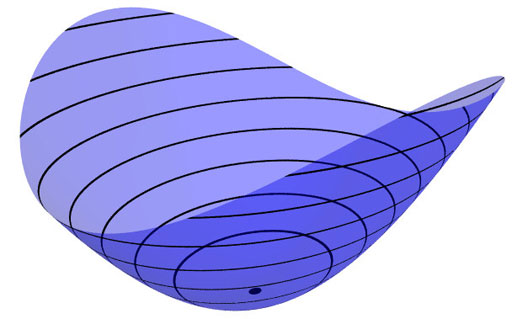Evaluation of polynomial functions in P gives us an algebra epimorphism $\mathbb{C}[X] \rightarrow \mathbb{C}$ from the coordinate ring of the variety $\mathbb{C}[X]$ onto $\mathbb{C}$ and the kernel of this map is the maximal ideal $\mathfrak{m}_P$ of
$\mathbb{C}[X]$ consisting of all functions vanishing in P.

Equivalently, we can view the point $P= \mathbf{spec}~\mathbb{C}[X]/\mathfrak{m}_P$ as the scheme corresponding to the quotient $\mathbb{C}[X]/\mathfrak{m}_P$. Call this the 0-th formal neighborhood of the point P.

This sounds pretty useless, but let us now consider higher-order formal neighborhoods. Call the affine scheme $\mathbf{spec}~\mathbb{C}[X]/\mathfrak{m}_P^{n+1}$ the n-th forml neighborhood of P, then the first neighborhood, that is with coordinate ring $\mathbb{C}[X]/\mathfrak{m}_P^2$ gives us tangent-information. Alternatively, it gives the best linear approximation of functions near P.
The second neighborhood $\mathbb{C}[X]/\mathfrak{m}_P^3$ gives us the best quadratic approximation of function near P, etc. etc.

These successive quotients by powers of the maximal ideal $\mathfrak{m}_P$ form a system of algebra epimorphisms

$\ldots \frac{\mathbb{C}[X]}{\mathfrak{m}_P^{n+1}} \rightarrow \frac{\mathbb{C}[X]}{\mathfrak{m}_P^{n}} \rightarrow \ldots \ldots \rightarrow \frac{\mathbb{C}[X]}{\mathfrak{m}_P^{2}} \rightarrow \frac{\mathbb{C}[X]}{\mathfrak{m}_P} = \mathbb{C}$

and its inverse limit $\underset{\leftarrow}{lim}~\frac{\mathbb{C}[X]}{\mathfrak{m}_P^{n}} = \hat{\mathcal{O}}_{X,P}$ is the completion of the local ring in P and contains all the infinitesimal information (to any order) of the variety X in a neighborhood of P. That is, this completion $\hat{\mathcal{O}}_{X,P}$ contains all information that P can see of the variety X.

In case P is a smooth point of X, then X is a manifold in a neighborhood of P and then this completion
$\hat{\mathcal{O}}_{X,P}$ is isomorphic to the algebra of formal power series $\mathbb{C}[[ x_1,x_2,\ldots,x_d ]]$ where the $x_i$ form a local system of coordinates for the manifold X near P.

Right, after this lengthy recollection, back to our question what does the monster see of the modular group? Well, we have an algebra epimorphism

$\pi~:~\mathbb{C} PSL_2(\mathbb{Z}) \rightarrow \mathbb{C} \mathbb{M}$

and in analogy with the commutative case, all information the Monster can gain from the modular group is contained in the $\mathfrak{m}$-adic completion

$\widehat{\mathbb{C} PSL_2(\mathbb{Z})}_{\mathfrak{m}} = \underset{\leftarrow}{lim}~\frac{\mathbb{C} PSL_2(\mathbb{Z})}{\mathfrak{m}^n}$

where $\mathfrak{m}$ is the kernel of the epimorphism $\pi$ sending the two free generators of the modular group $PSL_2(\mathbb{Z}) = C_2 \ast C_3$ to the permutations g and h determined by the dessin of the pentagonal tiling of the Monster’s empire.

As it is a hopeless task to determine the Monster-empire explicitly, it seems even more hopeless to determine the kernel $\mathfrak{m}$ let alone the completed algebra… But, (surprise) we can compute $\widehat{\mathbb{C} PSL_2(\mathbb{Z})}_{\mathfrak{m}}$ as explicitly as in the commutative case we have $\hat{\mathcal{O}}_{X,P} \simeq \mathbb{C}[[ x_1,x_2,\ldots,x_d ]]$ for a point P on a manifold X.

Here the details : the quotient $\mathfrak{m}/\mathfrak{m}^2$ has a natural structure of $\mathbb{C} \mathbb{M}$-bimodule. The group-algebra of the monster is a semi-simple algebra, that is, a direct sum of full matrix-algebras of sizes corresponding to the dimensions of the irreducible monster-representations. That is,

$\mathbb{C} \mathbb{M} \simeq \mathbb{C} \oplus M_{196883}(\mathbb{C}) \oplus M_{21296876}(\mathbb{C}) \oplus \ldots \ldots \oplus M_{258823477531055064045234375}(\mathbb{C})$

with exactly 194 components (the number of irreducible Monster-representations). For any $\mathbb{C} \mathbb{M}$-bimodule $M$ one can form the tensor-algebra

$T_{\mathbb{C} \mathbb{M}}(M) = \mathbb{C} \mathbb{M} \oplus M \oplus (M \otimes_{\mathbb{C} \mathbb{M}} M) \oplus (M \otimes_{\mathbb{C} \mathbb{M}} M \otimes_{\mathbb{C} \mathbb{M}} M) \oplus \ldots \ldots$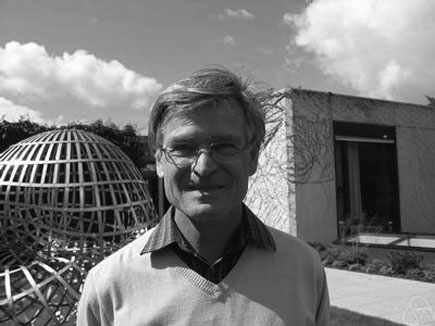and applying the formal neighborhood theorem for formally smooth algebras (such as $\mathbb{C} PSL_2(\mathbb{Z})$) due to Joachim Cuntz (left) and Daniel Quillen (right) we have an isomorphism of algebras

$\widehat{\mathbb{C} PSL_2(\mathbb{Z})}_{\mathfrak{m}} \simeq \widehat{T_{\mathbb{C} \mathbb{M}}(\mathfrak{m}/\mathfrak{m}^2)}$

where the right-hand side is the completion of the tensor-algebra (at the unique graded maximal ideal) of the $\mathbb{C} \mathbb{M}$-bimodule $\mathfrak{m}/\mathfrak{m}^2$, so we’d better describe this bimodule explicitly.

Okay, so what’s a bimodule over a semisimple algebra of the form $S=M_{n_1}(\mathbb{C}) \oplus \ldots \oplus M_{n_k}(\mathbb{C})$? Well, a simple S-bimodule must be either (1) a factor $M_{n_i}(\mathbb{C})$ with all other factors acting trivially or (2) the full space of rectangular matrices $M_{n_i \times n_j}(\mathbb{C})$ with the factor $M_{n_i}(\mathbb{C})$ acting on the left, $M_{n_j}(\mathbb{C})$ acting on the right and all other factors acting trivially.

That is, any S-bimodule can be represented by a quiver (that is a directed graph) on k vertices (the number of matrix components) with a loop in vertex i corresponding to each simple factor of type (1) and a directed arrow from i to j corresponding to every simple factor of type (2).

That is, for the Monster, the bimodule $\mathfrak{m}/\mathfrak{m}^2$ is represented by a quiver on 194 vertices and now we only have to determine how many loops and arrows there are at or between vertices.

Using Morita equivalences and standard representation theory of quivers it isn’t exactly rocket science to determine that the number of arrows between the vertices corresponding to the irreducible Monster-representations $S_i$ and $S_j$ is equal to

$dim_{\mathbb{C}}~Ext^1_{\mathbb{C} PSL_2(\mathbb{Z})}(S_i,S_j)-\delta_{ij}$

Now, I’ve been wasting a lot of time already here explaining what representations of the modular group have to do with quivers (see for example here or some other posts in the same series) and for quiver-representations we all know how to compute Ext-dimensions in terms of the Euler-form applied to the dimension vectors.

Right, so for every Monster-irreducible $S_i$ we have to determine the corresponding dimension-vector $~(a_1,a_2;b_1,b_2,b_3)$ for the quiver

$\xymatrix{ & & & & \vtx{b_1} \\ \vtx{a_1} \ar[rrrru]^(.3){B_{11}} \ar[rrrrd]^(.3){B_{21}} \ar[rrrrddd]_(.2){B_{31}} & & & & \\ & & & & \vtx{b_2} \\ \vtx{a_2} \ar[rrrruuu]_(.7){B_{12}} \ar[rrrru]_(.7){B_{22}} \ar[rrrrd]_(.7){B_{23}} & & & & \\ & & & & \vtx{b_3}}$

Now the dimensions $a_i$ are the dimensions of the +/-1 eigenspaces for the order 2 element g in the representation and the $b_i$ are the dimensions of the eigenspaces for the order 3 element h. So, we have to determine to which conjugacy classes g and h belong, and from Wilson’s paper mentioned above these are classes 2B and 3B in standard Atlas notation.

So, for each of the 194 irreducible Monster-representations we look up the character values at 2B and 3B (see below for the first batch of those) and these together with the dimensions determine the dimension vector $~(a_1,a_2;b_1,b_2,b_3)$.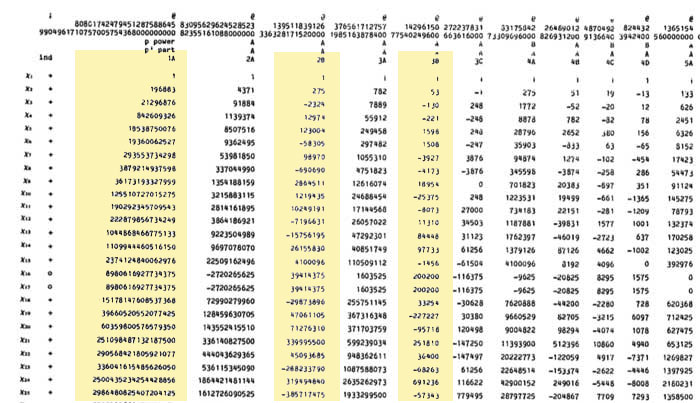For example take the 196883-dimensional irreducible. Its 2B-character is 275 and the 3B-character is 53. So we are looking for a dimension vector such that $a_1+a_2=196883, a_1-275=a_2$ and $b_1+b_2+b_3=196883, b_1-53=b_2=b_3$ giving us for that representation the dimension vector of the quiver above $~(98579,98304,65663,65610,65610)$.

Okay, so for each of the 194 irreducibles $S_i$ we have determined a dimension vector $~(a_1(i),a_2(i);b_1(i),b_2(i),b_3(i))$, then standard quiver-representation theory asserts that the number of loops in the vertex corresponding to $S_i$ is equal to

$dim(S_i)^2 + 1 – a_1(i)^2-a_2(i)^2-b_1(i)^2-b_2(i)^2-b_3(i)^2$

and that the number of arrows from vertex $S_i$ to vertex $S_j$ is equal to

$dim(S_i)dim(S_j) – a_1(i)a_1(j)-a_2(i)a_2(j)-b_1(i)b_1(j)-b_2(i)b_2(j)-b_3(i)b_3(j)$

This data then determines completely the $\mathbb{C} \mathbb{M}$-bimodule $\mathfrak{m}/\mathfrak{m}^2$ and hence the structure of the completion $\widehat{\mathbb{C} PSL_2}_{\mathfrak{m}}$ containing all information the Monster can gain from the modular group.

But then, one doesn’t have to go for the full regular representation of the Monster. Any faithful permutation representation will do, so we might as well go for the one of minimal dimension.

That one is known to correspond to the largest maximal subgroup of the Monster which is known to be a two-fold extension $2.\mathbb{B}$ of the Baby-Monster. The corresponding permutation representation is of dimension 97239461142009186000 and decomposes into Monster-irreducibles

$S_1 \oplus S_2 \oplus S_4 \oplus S_5 \oplus S_9 \oplus S_{14} \oplus S_{21} \oplus S_{34} \oplus S_{35}$

(in standard Atlas-ordering) and hence repeating the arguments above we get a quiver on just 9 vertices! The actual numbers of loops and arrows (I forgot to mention this, but the quivers obtained are actually symmetric) obtained were found after laborious computations mentioned in this post and the details I’ll make avalable here.

Anyone who can spot a relation between the numbers obtained and any other part of mathematics will obtain quantities of genuine (ie. non-Inbev) Belgian beer…

It’s been a while, so let’s include a recap : a (transitive) permutation representation of the modular group $\Gamma = PSL_2(\mathbb{Z})$ is determined by the conjugacy class of a cofinite subgroup $\Lambda \subset \Gamma$, or equivalently, to a dessin d’enfant. We have introduced a quiver (aka an oriented graph) which comes from a triangulation of the compactification of $\mathbb{H} / \Lambda$ where $\mathbb{H}$ is the hyperbolic upper half-plane. This quiver is independent of the chosen embedding of the dessin in the Dedeking tessellation. (For more on these terms and constructions, please consult the series Modular subgroups and Dessins d’enfants).

Why are quivers useful? To start, any quiver $Q$ defines a noncommutative algebra, the path algebra $\mathbb{C} Q$, which has as a $\mathbb{C}$-basis all oriented paths in the quiver and multiplication is induced by concatenation of paths (when possible, or zero otherwise). Usually, it is quite hard to make actual computations in noncommutative algebras, but in the case of path algebras you can just see what happens.

Moreover, we can also see the finite dimensional representations of this algebra $\mathbb{C} Q$. Up to isomorphism they are all of the following form : at each vertex $v_i$ of the quiver one places a finite dimensional vectorspace $\mathbb{C}^{d_i}$ and any arrow in the quiver
$$\xymatrix{\vtx{v_i} \ar[r]^a & \vtx{v_j}}$$ determines a linear map between these vertex spaces, that is, to $a$ corresponds a matrix in $M_{d_j \times d_i}(\mathbb{C})$. These matrices determine how the paths of length one act on the representation, longer paths act via multiplcation of matrices along the oriented path.

A necklace in the quiver is a closed oriented path in the quiver up to cyclic permutation of the arrows making up the cycle. That is, we are free to choose the start (and end) point of the cycle. For example, in the one-cycle quiver

$$\xymatrix{\vtx{} \ar[rr]^a & & \vtx{} \ar[ld]^b \\ & \vtx{} \ar[lu]^c &}$$

the basic necklace can be represented as $abc$ or $bca$ or $cab$. How does a necklace act on a representation? Well, the matrix-multiplication of the matrices corresponding to the arrows gives a square matrix in each of the vertices in the cycle. Though the dimensions of this matrix may vary from vertex to vertex, what does not change (and hence is a property of the necklace rather than of the particular choice of cycle) is the trace of this matrix. That is, necklaces give complex-valued functions on representations of $\mathbb{C} Q$ and by a result of Artin and Procesi there are enough of them to distinguish isoclasses of (semi)simple representations! That is, linear combinations a necklaces (aka super-potentials) can be viewed, after taking traces, as complex-valued functions on all representations (similar to character-functions).

In physics, one views these functions as potentials and it then interested in the points (representations) where this function is extremal (minimal) : the vacua. Clearly, this does not make much sense in the complex-case but is relevant when we look at the real-case (where we look at skew-Hermitian matrices rather than all matrices). A motivating example (the Yang-Mills potential) is given in Example 2.3.2 of Victor Ginzburg’s paper Calabi-Yau algebras.

Let $\Phi$ be a super-potential (again, a linear combination of necklaces) then our commutative intuition tells us that extrema correspond to zeroes of all partial differentials $\frac{\partial \Phi}{\partial a}$ where $a$ runs over all coordinates (in our case, the arrows of the quiver). One can make sense of differentials of necklaces (and super-potentials) as follows : the partial differential with respect to an arrow $a$ occurring in a term of $\Phi$ is defined to be the path in the quiver one obtains by removing all 1-occurrences of $a$ in the necklaces (defining $\Phi$) and rearranging terms to get a maximal broken necklace (using the cyclic property of necklaces). An example, for the cyclic quiver above let us take as super-potential $abcabc$ (2 cyclic turns), then for example

$\frac{\partial \Phi}{\partial b} = cabca+cabca = 2 cabca$

(the first term corresponds to the first occurrence of $b$, the second to the second). Okay, but then the vacua-representations will be the representations of the quotient-algebra (which I like to call the vacualgebra)

$\mathcal{U}(Q,\Phi) = \frac{\mathbb{C} Q}{(\partial \Phi/\partial a, \forall a)}$

which in ‘physical relevant settings’ (whatever that means…) turn out to be Calabi-Yau algebras.

But, let us return to the case of subgroups of the modular group and their quivers. Do we have a natural super-potential in this case? Well yes, the quiver encoded a triangulation of the compactification of $\mathbb{H}/\Lambda$ and if we choose an orientation it turns out that all ‘black’ triangles (with respect to the Dedekind tessellation) have their arrow-sides defining a necklace, whereas for the ‘white’ triangles the reverse orientation makes the arrow-sides into a necklace. Hence, it makes sense to look at the cubic superpotential $\Phi$ being the sum over all triangle-sides-necklaces with a +1-coefficient for the black triangles and a -1-coefficient for the white ones. Let’s consider an index three example from a previous post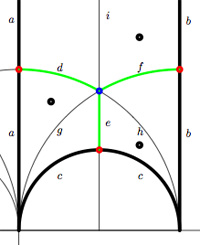$$\xymatrix{& & \rho \ar[lld]_d \ar[ld]^f \ar[rd]^e & \\ i \ar[rrd]_a & i+1 \ar[rd]^b & & \omega \ar[ld]^c \\ & & 0 \ar[uu]^h \ar@/^/[uu]^g \ar@/_/[uu]_i &}$$

In this case the super-potential coming from the triangulation is

$\Phi = -aid+agd-cge+che-bhf+bif$

and therefore we have a noncommutative algebra $\mathcal{U}(Q,\Phi)$ associated to this index 3 subgroup. Contrary to what I believed at the start of this series, the algebras one obtains in this way from dessins d’enfants are far from being Calabi-Yau (in whatever definition). For example, using a GAP-program written by Raf Bocklandt Ive checked that the growth rate of the above algebra is similar to that of $\mathbb{C}[x]$, so in this case $\mathcal{U}(Q,\Phi)$ can be viewed as a noncommutative curve (with singularities).

However, this is not the case for all such algebras. For example, the vacualgebra associated to the second index three subgroup (whose fundamental domain and quiver were depicted at the end of this post) has growth rate similar to that of $\mathbb{C} \langle x,y \rangle$…

I have an outlandish conjecture about the growth-behavior of all algebras $\mathcal{U}(Q,\Phi)$ coming from dessins d’enfants : the algebra sees what the monodromy representation of the dessin sees of the modular group (or of the third braid group).
I can make this more precise, but perhaps it is wiser to calculate one or two further examples…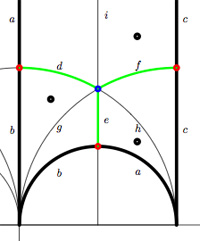We have associated to a subgroup of the modular group $PSL_2(\mathbb{Z})$ a quiver (that is, an oriented graph). For example, one verifies that the fundamental domain of the subgroup $\Gamma_0(2)$ (an index 3 subgroup) is depicted on the right by the region between the thick lines with the identification of edges as indicated. The associated quiver is then

$\xymatrix{i \ar[rr]^a \ar[dd]^b & & 1 \ar@/^/[ld]^h \ar@/_/[ld]_i \\ & \rho \ar@/^/[lu]^d \ar@/_/[lu]_e \ar[rd]^f & \\ 0 \ar[ru]^g & & i+1 \ar[uu]^c}$

The corresponding “dessin d’enfant” are the green edges in the picture. But, the red dot on the left boundary is identied with the red dot on the lower circular boundary, so the dessin of the modular subgroup $\Gamma_0(2)$ is

$\xymatrix{| \ar@{-}[r] & \bullet \ar@{-}@/^8ex/[r] \ar@{-}@/_8ex/[r] & -}$

Here, the three red dots (all of them even points in the Dedekind tessellation) give (after the identification) the two points indicated by a $\mid$ whereas the blue dot (an odd point in the tessellation) is depicted by a $\bullet$. There is another ‘quiver-like’ picture associated to this dessin, a quilt of the modular subgroup $\Gamma_0(2)$ as studied by John Conway and Tim Hsu.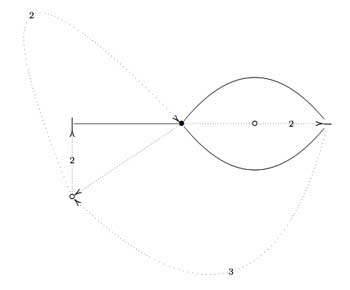On the left, a quilt-diagram copied from Hsu’s book Quilts : central extensions, braid actions, and finite groups, exercise 3.3.9. This ‘quiver’ has also 5 vertices and 7 arrows as our quiver above, so is there a connection?

A quilt is a gadget to study transitive permutation representations of the braid group $B_3$ (rather than its quotient, the modular group $PSL_2(\mathbb{Z}) = B_3/\langle Z \rangle$ where $\langle Z \rangle$ is the cyclic center of $B_3$. The $Z$-stabilizer subgroup of all elements in a transitive permutation representation of $B_3$ is the same and hence of the form $\langle Z^M \rangle$ where M is called the modulus of the representation. The arrow-data of a quilt, that is the direction of certain edges and their labeling with numbers from $\mathbb{Z}/M \mathbb{Z}$ (which have to satisfy some requirements, the flow rules, but more about that another time) encode the Z-action on the permutation representation. The dimension of the representation is $M \times k$ where $k$ is the number of half-edges in the dessin. In the above example, the modulus is 5 and the dessin has 3 (half)edges, so it depicts a 15-dimensional permutation representation of $B_3$.

If we forget the Z-action (that is, the arrow information), we get a permutation representation of the modular group (that is a dessin). So, if we delete the labels and directions on the edges we get what Hsu calls a modular quilt, that is, a picture consisting of thick edges (the dessin) together with dotted edges which are called the seams of the modular quilt. The modular quilt is merely another way to depict a fundamental domain of the corresponding subgroup of the modular group. For the above example, we have the indicated correspondences between the fundamental domain of $\Gamma_0(2)$ in the upper half-plane (on the left) and as a modular quilt (on the right)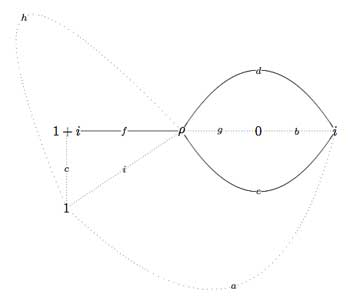That is, we can also get our quiver (or its opposite quiver) from the modular quilt by fixing the orientation of one 2-cell. For example, if we fix the orientation of the 2-cell $\vec{fch}$ we get our quiver back from the modular quilt$\xymatrix{i \ar[rr]^a \ar[dd]^b & & 1 \ar@/^/[ld]^h \ar@/_/[ld]_i \\ & \rho \ar@/^/[lu]^d \ar@/_/[lu]_e \ar[rd]^f & \\ 0 \ar[ru]^g & & i+1 \ar[uu]^c}$

This shows that the quiver (or its opposite) associated to a (conjugacy class of a) subgroup of $PSL_2(\mathbb{Z})$ does not depend on the choice of embedding of the dessin (or associated cuboid tree diagram) in the upper half-plane. For, one can get the modular quilt from the dessin by adding one extra vertex for every connected component of the complement of the dessin (in the example, the two vertices corresponding to 0 and 1) and drawing a triangulation from them (the dotted lines or ‘seams’).

Last time we have that that one can represent (the conjugacy class of) a finite index subgroup of the modular group $\Gamma = PSL_2(\mathbb{Z})$ by a Farey symbol or by a dessin or by its fundamental domain. Today we will associate a quiver to it.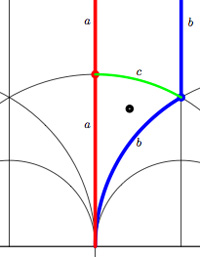For example, the modular group itself is represented by the Farey symbol
$$\xymatrix{\infty \ar@{-}[r]_{\circ} & 0 \ar@{-}[r]_{\bullet} & \infty}$$ or by its dessin (the green circle-edge) or by its fundamental domain which is the region of the upper halfplane bounded by the red and blue vertical boundaries. Both the red and blue boundary consist of TWO edges which are identified with each other and are therefore called a and b. These edges carry a natural orientation given by circling counter-clockwise along the boundary of the marked triangle (or clockwise along the boundary of the upper unmarked triangle having $\infty$ as its third vertex). That is the edge a is oriented from $i$ to $0$ (or from $i$ to $\infty$) and the edge b is oriented from $0$ to $\rho$ (or from $\infty$ to $\rho$) and the green edge c (which is an inner edge so carries no identifications) from $\rho$ to $i$. That is, the fundamental region consists of two triangles, glued together along their boundary which is the oriented cycle $\vec{abc}$ consistent with the fact that the compactification of $\mathcal{H}/\Gamma$ is the 2-sphere $S^2 = \mathbb{P}^1_{\mathbb{C}}$. Under this identification the triangle-boundary abc can be seen to circle the equator whereas the top triangle gives the upper half sphere and the lower triangle the lower half sphere. Emphasizing the orientation we can depict the triangle-boundary as the quiver

$$\xymatrix{i \ar[rd]_a & & \rho \ar[ll]_c \\ & 0 \ar[ru]_b}$$

embedded in the 2-sphere. Note that quiver is just a fancy name for an oriented graph…Okay, let’s look at the next case, that of the unique index 2 subgroup $\Gamma_2$ represented by the Farey symbol $$\xymatrix{\infty \ar@{-}[r]_{\bullet} & 0 \ar@{-}[r]_{\bullet} & \infty}$$ or the dessin (the two green edges) or by its fundamental domain consisting of the 4 triangles where again the left and right vertical boundaries are to be identified in parts.

That is we have 6 edges on the 2-sphere $\mathcal{H}/\Gamma_2 = S^2$ all of them oriented by the above rule. So, for example the lower-right triangle is oriented as $\vec{cfb}$. To see how this oriented graph (the quiver) is embedded in $S^2$ view the big lower region (cdab) as the under hemisphere and the big upper region (abcd) as the upper hemisphere. So, the two green edges together with a and b are the equator and the remaining two yellow edges form the two parts of a bigcircle connecting the north and south pole. That is, the graph are the cut-lines if we cut the sphere in 4 equal parts. The corresponding quiver-picture is

$$\xymatrix{& i \ar@/^/[dd]^f \ar@/_/[dd]_e & \\ \rho^2 \ar[ru]^d & & \rho \ar[lu]_c \\ & 0 \ar[lu]^a \ar[ru]_b &}$$As a mental check, verify that the index 3 subgroup determined by the Farey symbol $$\xymatrix{\infty \ar@{-}[r]_{\circ} & 0 \ar@{-}[r]_{\circ} & 1 \ar@{-}[r]_{\circ} & \infty}$$ , whose fundamental domain with identifications is given on the left, has as its associated quiver picture

$$\xymatrix{& & \rho \ar[lld]_d \ar[ld]^f \ar[rd]^e & \\ i \ar[rrd]_a & i+1 \ar[rd]^b & & \omega \ar[ld]^c \\ & & 0 \ar[uu]^h \ar@/^/[uu]^g \ar@/_/[uu]_i &}$$whereas the index 3 subgroup determined by the Farey symbol $$\xymatrix{\infty \ar@{-}[r]_{1} & 0 \ar@{-}[r]_{1} & 1 \ar@{-}[r]_{\circ} & \infty}$$, whose fundamental domain with identifications is depicted on the right, has as its associated quiver

$$\xymatrix{i \ar[rr]^a \ar[dd]^b & & 1 \ar@/^/[ld]^h \ar@/_/[ld]_i \\ & \rho \ar@/^/[lu]^d \ar@/_/[lu]_e \ar[rd]^f & \\ 0 \ar[ru]^g & & i+1 \ar[uu]^c}$$

Next time, we will use these quivers to define superpotentials…